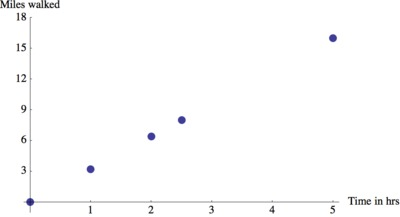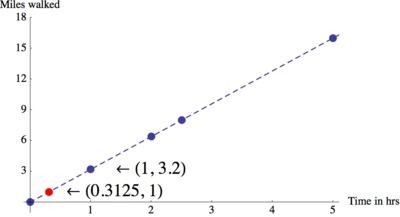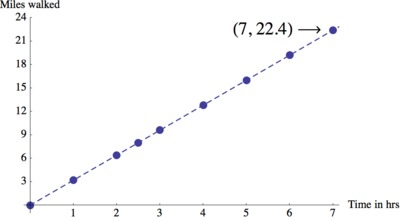# Walk-a-thon 2

Alignments to Content Standards: 7.RP.A.2

Julianna participated in a walk-a-thon to raise money for cancer research. She recorded the total distance she walked at several different points in time, but a few of the entries got smudged and can no longer be read. The times and distances that can still be read are listed in the table below.

1. Assume Julianna walked at a constant speed. Complete the table and plot Juliannaâ€™s progress in the coordinate plane.
2. What was Juliannaâ€™s walking rate in miles per hour? How long did it take Julianna to walk one mile? Where do you see this information on the graph?
3. Write an equation for the distance $d$, in miles, that Julianna walked in $n$ hours.
4. Next year Julianna is planning to walk for seven hours. If she walks at the same speed next year, how many miles will she walk?
Time in hrs Miles walked
1 Â
2 6.4
Â  8
5 Â

## IM Commentary

The purpose of this task is for students to translate information about a context involving constant speed into information presented in a table and to find the time it takes to travel a unit distance as well as the distance traveled per unit time. Students then have to translate the information to equations and graphs and then use these mathematical tools to make predictions about the future.

If we only used integer values, the first few parts of this task could come from a 6th grade task. In fact, a 6th grade version of this task is 6.RP Walk-a-thon.

In 7th grade, students work with rational numbers, they are also expected to identify the unit rate as a coordinate of a point on the graph $(1,r)$ and to write an equation that relates the quantities that are in a proportional relationship. The task directly addresses parts (b), (c), and (d) of 7.RP.A.2, and it indirectly addresses part (a) because it requires students to work in the opposite direction. Part (a) requires more complex reasoning than simply producing a table for two quantities in a proportional relationship because it requires them to use information in the table to generate other specific ordered pairs rather than writing any correct ordered pairs they want.

The teacher may wish to explicitly ask students to answer part (d) using all three representations as tools and ask them to comment on the usefulness and limitations of each representation. In this case, the task could be used to launch a whole-class discussion about the connections between tables, equations, and graphs.

The Standards for Mathematical Practice focus on the nature of the learning experiences by attending to the thinking processes and habits of mind that students need to develop in order to attain a deep and flexible understanding of mathematics. Certain tasks lend themselves to the demonstration of specific practices by students. The practices that are observable during exploration of a task depend on how instruction unfolds in the classroom. While it is possible that tasks may be connected to several practices, the commentary will spotlight one practice connection in depth. Possible secondary practice connections may be discussed but not in the same degree of detail.

Mathematical Practice 5, â€œUse appropriate tools strategically,â€ emphasizes a studentâ€™s ability to consider a toolâ€™s usefulness and constraints as well as knowing how to use it appropriately. During this task, students engage in MP.5 by considering the connections among tables, graphs, and equations (tools) to find the time it takes to travel a unit distance in addition to the distance traveled per unit time. They extend their understanding by using these same tools to predict how many miles Julianna will walk next year if she maintains the same speed. Although this particular task directs students to use specific tools, it does not prevent them from trying additional tools such as a double number line. This lends itself nicely into a class discussion regarding the pros and cons of using each of these tools.

## Solution

1. We see that the only complete time-distance pair indicates that Julianna walked 6.4 miles in 2 hours. If she walked at a constant speed, we can conclude that Julianna walked 3.2 miles in 1 hour. Using this speed, we can find the remaining values in the table. The easier entry to complete is finding out how far Julianna walked in 5 hours: $5 \text{ hours} \cdot 3.2 \text{ miles/hour} = 16 \text{ miles}$. To find out how long it took to walk 8 hours we can either notice that 8 is half of 16, so it took half the time to walk half the distance or we can divide 8 miles by 3.2 miles per hour to find that it took 2.5 hours (but this calculation is not obvious).

We get the following table.

Time in hrs Miles walked
1 3.2
2 6.4
2.5 8
5 16

Let's plot her progress in the coordinate plane by having her time, in hours, be represented on the horizontal axis, and the number of miles she walked be represented on the vertical axis. Using the rows in the table above as our coordinate points, we get the following graph.2. We found in part (a) that Julianna walks 3.2 miles in 1 hour.

We find the part of the graph that holds this information, i.e. the point with coordinates (1,3.2).We can also use the graph to estimate out how long it takes Julianna to walk one mile. Since Julianna walks at a constant rate, we can connect the points with a line to visualize how far she has walked at any time. This allows us to see that it took a little bit less than $\frac13$ of an hour to walk 1 mile.

To find the exact value we can use that it takes Julianna 1 hour to walk 3.2 miles or $\frac{1 \text{ hour}}{3.2 \text{ miles}}$. We would like to know how much time it took to walk 1 mile or $\frac{\text{?? hours}}{1 \text{ mile}}$. Starting with $\frac{1 \text{ hour}}{3.2 \text{ mile}}$ we have $$\frac{1 \text{ hour}\cdot\frac{1}{3.2}}{3.2 \text{ mile}\frac{1}{3.2}} = \frac{0.3125\text{ hour}}{1 \text{ mile}}$$

We can also use a double number line to find the answer.If we divide the 3.2 mile line segment into 16 pieces of 0.2 mile length we can see that we have 5 of the 0.2 mile pieces to get to 1 mile. We also divide the 1 hour line segment into 16 equal pieces, each of which has length 1/16 mile. We see that 5 of the 1/16 mile pieces will get us to line up with the 1 mile mark. Therefore, in 1 hour Julianna walks $5/16=0.3125$ mile.

3. Julianna walks 3.2 miles every hour, so if we let $n$ be the number of hours walked, we have $d=3.2n$.

4. We have three different representations that we can use to find out how far Julianna would walk in 7 hours. We found in parts (a) and (b) that Juliannaâ€™s rate of travel was 3.2 miles per hour. If this stayed constant, we can find how many miles she would walk in 7 hours by extending our table.

Time in hrs Miles walked
1 3.2
2 6.4
2.5 8
5 16
6 19.2
7 22.4

Thus, we see that in 7 hours, Julianna will walk 22.4 miles at that rate.

We can also use the equation $d=3.2n$ with $n=7$ to find that $d=3.2\cdot7=22.4$ miles.

Finally, we can extend the graph to 7 hours on the horizontal axis to estimate that the line passes through the point (7,22.4), although reading off the exact distance is not very easy.